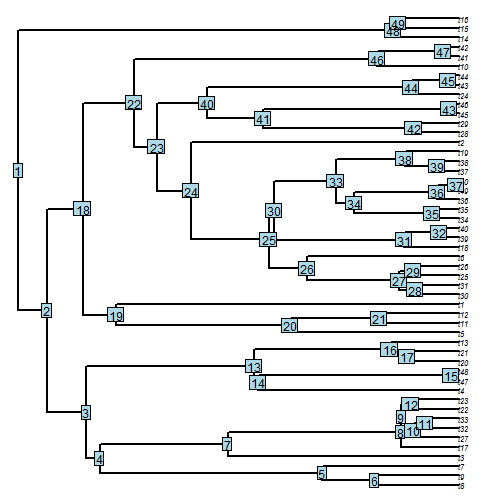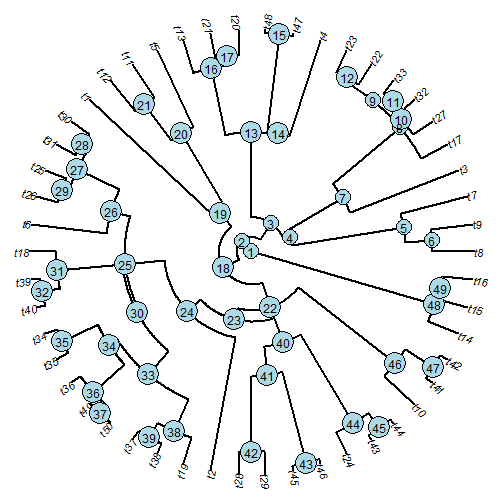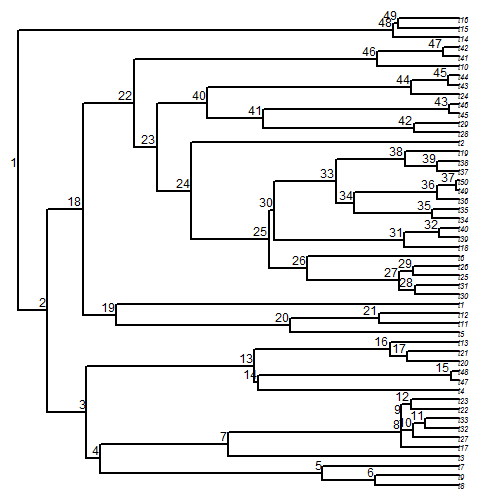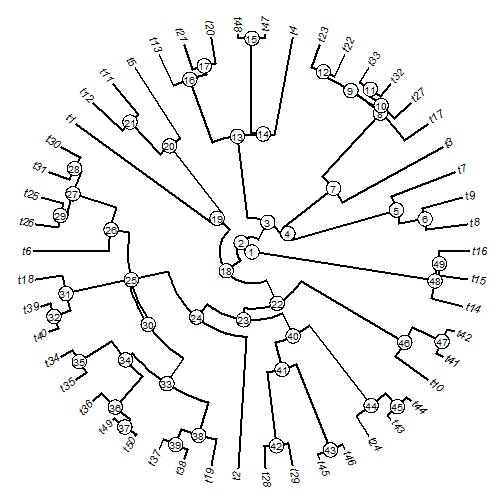## Tuesday, February 21, 2017

### More on labeling nodes using `ape::nodelabels` & phytools

The colleague question that inspired my recent post on interactively adding node labels to a tree was actually must simpler & is as follows:

“A student of mine has a 250 taxon tree that we want to number the nodes for on the figure, starting with "1” (preferably the root, but we can work with most any logical system) through 249. Is there an easy way in phytools?“

This is indeed pretty straightforward. I'm going to work with a small tree of 50 taxa - just to not overwhelm the size of plot window I can create on my blog - but the principle is identical.

First of all - we can easily do it with `ape::nodelabels`.

``````library(ape)
tree
``````
``````##
## Phylogenetic tree with 50 tips and 49 internal nodes.
##
## Tip labels:
##  t8, t9, t7, t3, t17, t27, ...
##
## Rooted; includes branch lengths.
``````
``````plot(tree,no.margin=TRUE,edge.width=2,cex=0.7)
nodelabels(text=1:tree\$Nnode,node=1:tree\$Nnode+Ntip(tree))
``````There are many different options with `nodelabels`. For instance, we can plot our tree in `"fan"` style, and our node labels within circles:

``````plot(tree,no.margin=TRUE,edge.width=2,type="fan",cex=0.9)
nodelabels(text=1:tree\$Nnode,node=1:tree\$Nnode+Ntip(tree),frame="circle")
``````We don't need to use frames around our labels. We can instead offset them from the plotted edges:

``````plot(tree,no.margin=TRUE,edge.width=2,cex=0.7)
nodelabels(text=1:tree\$Nnode,node=1:tree\$Nnode+Ntip(tree),
``````Equally well, we could employ the new function that I have just added to the phytools package. For instance:

``````library(phytools)
packageVersion("phytools")
``````
``````##  '0.5.77'
``````
``````plotTree(tree,type="fan",fsize=0.9,ftype="i")
labelnodes(text=1:tree\$Nnode,node=1:tree\$Nnode+Ntip(tree),
interactive=FALSE,circle.exp=0.9,cex=0.8)
``````I like that.

1.Is it possible to replace the nodelabel numbers with names? for example, to name genera and families?

1.Sure. In that case the argument text is a vector of the text strings you want to plot, while node gives a vector of the corresponding node numbers.

2.How can I retrieve node numbers corresponding to labels stored in tree\$node.label? I have all my nodes labeled in the tree object, but I want to label only some of them as defined in a vector. e.g. tree with 3 nodes labeled A, B, C - I want to label only A and C. I know that you can first display node numbers and then manually insert them to the nodelabels(), but I have hundreds of complicated trees in which I want to label corresponding nodes with same labels. Thanks

2.Dear Liam, I have been using for some thing these amazing suit of functions, thank you! I have a question, if its possible. Everytime I root the tree like midpoint, and plot the node.labels to show the support, is also ploting the "Root" word. Any tip of how to avoid this ploting? Thanks!
- Felipe

1.Hi Felipe. Try using the argument node in ape::nodelabels to indicate the nodes you want to label - but then leave out the root! The root node is always Ntip(tree)+1.
- Liam

3.Thanks Liam!!

Note: due to the very large amount of spam, all comments are now automatically submitted for moderation.